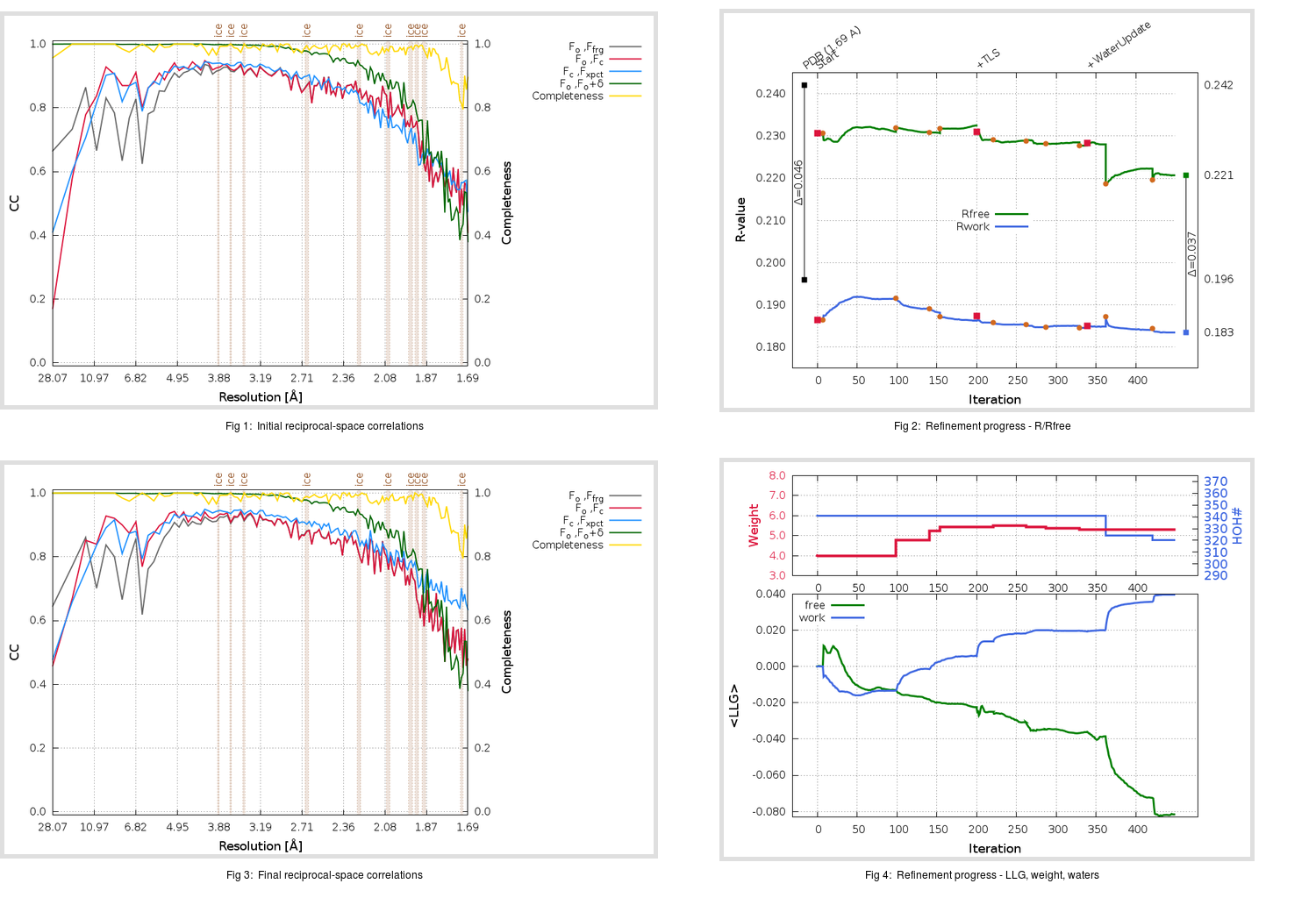Content:

## Deposited

` `
 Date deposited Date data collection Resolution R, Rfree 20200315 20200305 1.69 0.1930 0.2420

Molprobity (CCP4 7.0 version) summary:

```Ramachandran outliers =   0.33 %
favored =  98.01 %
Rotamer outliers      =   0.74 %
C-beta deviations     =     0
Clashscore            =   4.76
RMS(bonds)            =   0.0126
RMS(angles)           =   1.76
MolProbity score      =   1.25
Resolution            =   1.69
R-work                =   0.1930
R-free                =   0.2420
```

```Number of waters      =   341

<B> (all atoms) =   27.66 ( sd =    9.27 ) for       2789 non-hydrogen atoms
<B>   (protein) =   26.41 ( sd =    8.64 ) for       2416 non-hydrogen atoms
<B>     (water) =   35.96 ( sd =    9.62 ) for        341 non-hydrogen atoms
<B>    (others) =   34.76 ( sd =   11.02 ) for         32 non-hydrogen atoms

B min/max       (all non-hydrogen atoms) =   15.26 /   76.75
B min/max   (protein non-hydrogen atoms) =   15.78 /   74.55
B min/max     (water non-hydrogen atoms) =   15.26 /   76.75
B min/max     (other non-hydrogen atoms) =   25.60 /   50.37
```

## BUSTER (re-)refinement

` `

Molprobity (CCP4 7.0 version) summary:

```Ramachandran outliers =   0.33 %
favored =  98.68 %
Rotamer outliers      =   0.74 %
C-beta deviations     =     0
Clashscore            =   3.73
RMS(bonds)            =   0.0112
RMS(angles)           =   1.60
MolProbity score      =   1.16
Resolution            =   1.69
R-work                =   0.1835
R-free                =   0.2207
```

```Number of waters      =   320

<B> (all atoms) =   27.76 ( sd =    8.58 ) for       2768 non-hydrogen atoms
<B>   (protein) =   26.38 ( sd =    7.50 ) for       2416 non-hydrogen atoms
<B>     (water) =   37.42 ( sd =    9.70 ) for        320 non-hydrogen atoms
<B>    (others) =   34.29 ( sd =   10.77 ) for         32 non-hydrogen atoms

B min/max       (all non-hydrogen atoms) =   15.54 /   71.48
B min/max   (protein non-hydrogen atoms) =   16.24 /   70.30
B min/max     (water non-hydrogen atoms) =   15.54 /   71.48
B min/max     (other non-hydrogen atoms) =   24.31 /   47.63
```

Refinement progression:Results:

` `
 File Remark 5RFI_aB_refine.01_03_refine.pdb.gz exact refinement commands are in header 5RFI_aB_refine.01_03_refine.mtz.gz including original deposited data and several re-refinement map coefficients 5RFI_aB_refine.01_03_BUSTER_model.cif.gz including any non-standard compound restraints 5RFI_aB_refine.01_03_BUSTER_refln.cif.gz